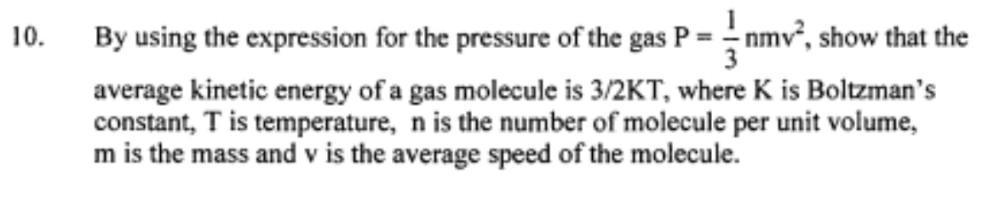Question:

# 10. By using the expression for the pressure of the gas P = 5nmv”, show that the average kinetic energy of a gas molecule is 3/210. By using the expression for the pressure of the gas P = 5nmv”, show that the average kinetic energy of a gas molecule is 3/2KT, where K is Boltzman's constant, T is temperature, n is the number of molecule per unit volume, m is the mass and v is the average speed of the molecule.